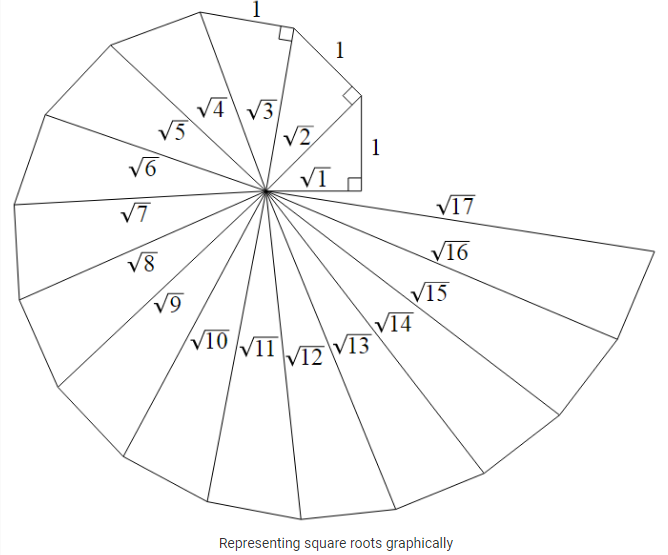Jet Set Go! All about Aeroplanes Jet Set Go! All about Aeroplanes

# Square Root of 120

The square root of 120 is the value which when multiplied by itself gives the result as 120. signifies the reverse of the square of a number. Basically, the square root of a number say ‘n’, is another number say ‘m’, in such a way that when m is multiplied to itself or when we take the square of m, it gives number ‘n’.

The square root of the rational number, 120 gives a decimal number and not a whole number. It is because the number 120 doesn’t have proper factors. Usually, by the method of prime factorization, we get the set of numbers that are multiplied together to get the initial number.

For all non-negative real numbers, we can find a unique non-negative square root, which is called the principal square root. Therefore, the square root of 120 gives us a unique non-negative number. It is denoted as √120, where √ is called radical sign or radix. The number 120, whose square has to be derived is called radicand. Or we can say the term or the number underneath of the radical sign is specified as radicand.

## Square Root of 120 Value

 Square Root of 120 (√120) = 10.954

## Simplification of Square Root of 120

The value of the square root of 120 is 10.954451150103. Let us learn how to find out its value. As discussed, when a number is multiplied by itself, it gives a value whose square root can be taken. Like if write the square of a number equal to another b, we get,

b = a2

Now if we have to take the square root of b, we get,

√b = √a2

So, the root cancels the square or un-square it. Therefore we get,

√b = a

Similarly, we can find the square root of 120 here, by writing its prime factors first.

We can write, 120 = 2 × 2 × 2 × 3 × 5

Taking out the root means, taking out the pair of numbers present underneath the radix.

Therefore, the square root of 120, √120 = √(2 × 2 × 2 × 3 × 5)

⇒ √120 = 2 √(2 × 3 × 5) = 2√30

⇒ √120 = 2√30.

This is the radical form of √120. But to find the accurate value, we have to mention the value of √30. The value of the square of 30 is 5.477. Therefore,

√120 = 2 × 5.477 = 10.9544

You can use square root long division method to find the value of √30. This method gives the perfect value for any square root. Apart from these are we have certain methods by the means of which we can find the square root of a given number.

We can represent the square roots in a graphical form.Properties of square roots

• The general form of representation of square root is √x = a, where x has two roots +a and -a.
•
• The principal square root function can be represented as f(x) = √x. It is used to map the area of the square to its side length.
•
• The square root of a perfect square gives non-fraction and non-decimal number.
•
• For all non-negative real numbers a and b,
• √ab = √a.√b
• The square root of a non-negative real number x, can be represented as,
• √x= x½

## Video Lessons on Square Roots

### Visualising square roots### Finding Square rootsDownload BYJU’S – The Learning App and learn to solve Maths problems with the help of interactive visuals.

Test your Knowledge on Square Root of 120